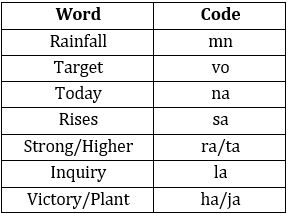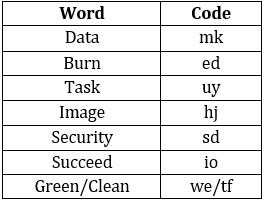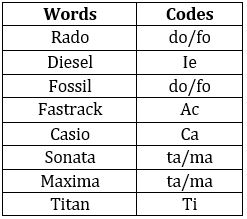Latest Banking jobs   »

# Reasoning Ability Quiz For SBI Clerk Prelims 2022- 9th September

Directions (1-5): Study the following information carefully and answer the questions given below:
In a certain code language
‘Rainfall today target’ is written as ‘mn vo na’,
‘Strong rises higher’ is written as ‘sa ra ta’,
‘Target rises inquiry’ is written as ‘la vo sa’,
‘Victory plant rainfall’ is written as ‘mn ha ja’.

Q1. What is the code for ‘rainfall’?
(a) ja
(b) ha
(c) mn
(d) na
(e) None of these

Q2. Which of the following may be the code for ‘victory higher target’?
(a) ja na ta
(b) vo ra ha
(c) vo ta mn
(d) Either (a) or (c)
(e) None of these

Q3. What is the code for ‘victory’?
(a) ja
(b) yo
(c) la
(d) ha
(e) Can’t be determined

Q4. What does ‘la’ stand for?
(a) strong
(b) inquiry
(c) today
(d) plant
(e) None of these

Q5. What is the code for ‘rises’?
(a) ja
(b) yo
(c) sa
(d) ha
(e)None of these

Directions (6-10): Study the following information carefully to answer the given questions.
In a certain code language,
“Data image burn task” is coded as “hj mk uy ed”
“Succeed security Data” is coded as “io mk sd”
“Burn security succeed” is coded as “io sd ed”
“Security task green clean” is coded as “sd we uy tf”

Q6. What is the code for ‘security’?
(a) io
(b) sd
(c) we
(d) uy
(e) None of these

Q7. What does code ‘ed’ stand for?
(a) Data
(c) Green
(d) Burn
(e) None of these

Q8. Which of the following code may be for ‘green image data’?
(a) ed sd stf
(b) io sd uy
(c) hj mk we
(d) mk we tf
(e) None of these

Q9. What is the code for ‘Image’?
(a) hj
(b) mk
(c) we
(d) sd
(e) None of these

Q10. What is the code for ‘Clean’?
(a) ed
(b) we
(c) tf
(d) Either (b) or (c)
(e) io

Directions (11-15): Study the following information carefully and answer the questions given below:
In a certain code language:
“Rado Diesel Fossil Fastrack” is coded as “ac ie fo do”
“Casio Sonata Maxima Diesel” is coded as “ie ca ta ma”
“Fastrack Diesel Casio Titan” is coded as “ti ie ca ac”

Q11. What is the code of “Casio” as per the given code language?
(a) ac
(b) ca
(c) ie
(d) fo
(e) None of these

Q12. What is the code of “Maxima” as per the given code language?
(a) ta
(b) ma
(c) ie
(d) ca
(e) can’t be determined

Q13. Which of the following word has code of “Fo” as per the given code language?
(a) Fossil
(b) Diesel
(d) Either (a) and (c)
(e) None of these

Q14. Which of the following words may have the code of “ta ti ca” as per the given code language?
(a) sonata titan maxima
(b) maxima casio diesel
(c) sonata casio titan
(d) maxima casio Fastrack
(e) None of these

Q15. What may be the code of “Diesel crystal” as per the given code language?
(a) ie ti
(b) al ie
(c) ma ie
(d) al ac
(e) None of these

Solutions

Solution (1-5):
Sol.S1. Ans. (c)
S2. Ans. (b)
S3. Ans. (e)
S4. Ans. (b)
S5. Ans. (c)

Solution (6-10):
Sol.S6. Ans. (b)
S7. Ans. (d)
S8. Ans. (c)
S9. Ans. (a)
S10. Ans. (d)

Solution (11-15):
Sol.S11. Ans(b)
S12. Ans(e)
S13. Ans(d)
S14. Ans(c)
S15. Ans(b)#### Congratulations!Download Hindu Review of October 2021: Free PDF